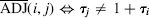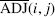## 2.8 MARKOV SCORING

Given: columnar transposition ciphertext y;

Find: the transposition width N and transposition τ.

Our plan is to test N as a possible width by computing a Marko score for the adjacency of columns in the ciphertext, assuming each of the N! transpositions of width N are equally likely to have been used.

Testing a width N is formulated as a hypotheses testing problem; for each pair (i, j) with ij, decide which of the two hypotheses is the most likely to be true., jth column is read from X immediately after theith column is read from X., jth column is not from X immediately after theith column is read from X.

When ADJ(i, j) is true, the ith and jth columns must be columns (k, k + 1) in X for some k with 0 ≤ k < n−1. As the N! transpositions τ have been chosen with equal probability, the a priori4 probabilities of the hypotheses ADJ(i, j) andareandThe ratio of these probabilities is the a priori odds of ADJ(i, j) over

The term ODDS has the same interpretation ...

Get Computer Security and Cryptography now with the O’Reilly learning platform.

O’Reilly members experience live online training, plus books, videos, and digital content from nearly 200 publishers.